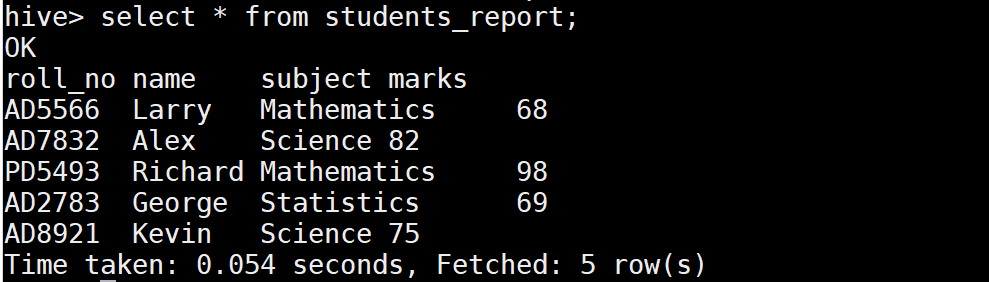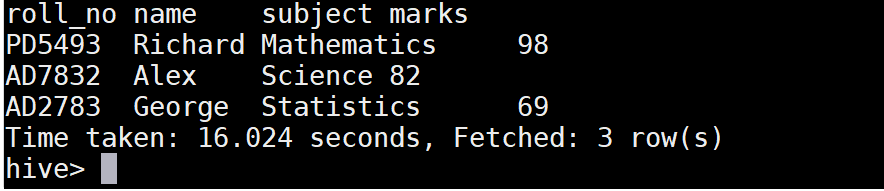# ROW_NUMBER() function in Hive

Row_number is one of the analytics function in Hive. It will assign the unique number(1,2,3…) for each row based on the column value that used in the OVER clause.

In addition, A partitioned By clause is used to split the rows into groups based on column value. Along with this, It allows to define the ORDER BY clause to sort the rows with in the group.

## Syntax of Row number in Hive

• Column_reference is the column name that used to divide the result set based on its value.
• Sort_expression is the another column name that used for sorting the result set with in each group.

## Example

Lets look at the Row_number() function with examples in Hive. We have a table students_report as below in Hive. This table contains the student marks details for each subject. The columns of this table are roll_no, name, subject and marks.Row number example in hive

From this table, we want to fetch the student details who got the highest marks in each subject. So the column reference for partition is subject and sort_expression can be defined using the marks column.

Lets write the query in Hive using the Row_number function as belowAssign row number in Hive

As we can see in the above image, the row number(1,2…) is assigned for each group based on subject. Since we defined the sorting expression as descending by marks, the row number 1 is assigned for the highest mark in each group. Then the subsequent row gets the next row number 2 in each group.

Lets fetch the row which has the rank as 1 so that we can get the topper list for each subject.

The Inner select query will return the result set with the row number. Since we want to fetch only the row which has the row number as 1, we have defined the derived table with the alias name as ranked_v for the result set of inner select query.

Then we have used the where condition in outer select query to fetch the required result.

## OutputFetch rows based on row number in Hive

Finally we got the student details who got the highest marks in each subject.